# Program to check Strong Number In Java

In this tutorial you will learn about the Program to check Strong Number In Java and its application with practical example.

In this tutorial, we will learn to create a Java Program to check Strong Numbers in Java using Java programming.

## Prerequisites

Before starting with this tutorial we assume that you are best aware of the following Java programming topics:

• Java Operators.
• Basic Input and Output function in Java.
• Class and Object in Java.
• Basic Java programming.
• If-else statements in Java.
• For loop in Java.

## Strong Number

A strong number is a number which sum of the factorial of the digits is equal to the number itself.
Example:-
145 = 1! + 4! + 5! = 1 + 24 + 120 = 145
So we can say that the 145 is a strong number.

## Program to check Strong Number In Java

In our program we will find given number is a strong number or not in java programming. We would first declared and initialized the required variables. Next, we would prompt user to input the age value .Later we calculate the age is valid for vote or not let’s have a look at the code.

## Output

Strong number.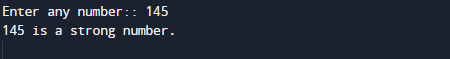Not a strong number.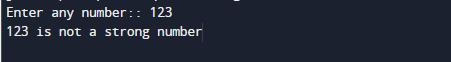In the above program, we have first declared and initialized a set variables required in the program.

• number= it will hold entered number
• shrt= it will hold value person short by how much.

After declaring variables in the next statement user will be prompted to enter a value and  which will be assigned to variable ‘number’.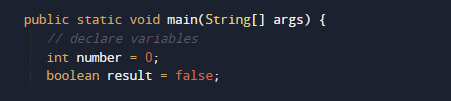And after that we calculate the value given number is strong or not by passing the number to user define function Strong(number) .within the function here first of all we are going to find factors of given number and also the factorial of each factor of a number.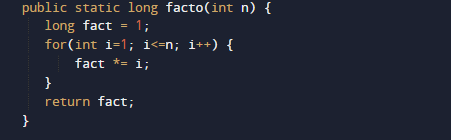after that we going add the values and check if given number and sum of factor of given area same as shown in image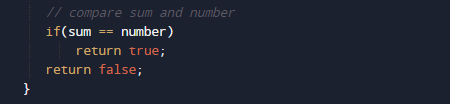If both are same number is strongIf not Not a strong number

In this tutorial we have learn about the Program to check Strong Number In Java and its application with practical example. I hope you will like this tutorial.